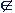## Operations on Sets

1. Union of Two Sets:

The Union of two sets A and B is an operation on the two sets.

It is a set that contains all of the elements of both the sets A and B.

The union of two sets A and B is denoted as AUB.

Set builder notation for the union of two sets A and B is

AUB = {x/x∈A or x∈B or x∈both A and B}

Example 1:

A = {1, 2, 3] and B = {2, 3, 4}.

The Union of the two sets A and B is

AUB = {1, 2, 3, 4}

Example 2:

X = {asia, america, australia} Y = {australia, europe, antarctica  } Z = {europe, india, asia }

X UYU Z = {asia, america, australia, europe, antarctica, india, u.s.a}

Note:

In the union of two sets, common elements need not be written more than one time. It is just enough to write common elements only once. In a set, an element is counted only once though it may be written any number of times.

For example the set A = {1, 1, 1} contains only one element, 1, though 1 is written 3 times in the set.

2. Intersection of two sets:

The intersection of two sets A and B is the set which contains the elements that are in both A and B.

It is denoted as A∩B

The intersection of two sets A and B in set builder form is

A∩B = { x/x∈A and x∈B}

Example 1:

If A = {1, 2, 3} and B = {3, 4, 5}, then what is A∩B?

A∩B = {1}

Example 2:

If A = {1, 2, 3} and B = {4, 5, 6}, then what is A∩B?

Since there is no element which is in both the set A and set B, therefore

A∩B = Φ

We can also write A∩B = { }

Example 3:

A = {factors of 8} B = {factors of 9}. What is A∩B?

A = {factors of 8} is the set {1, 2, 4, 8 ...} and

B = {factors of 9} is the set {1, 3, 6, 9...}

So, we can see that 1 is the only element which is in both set A and set B.

The only element common to both the sets A and B is 1. Therefore

A∩B = {1}

3. Difference of two sets:

Let A and B be two sets. Then the difference of the two sets A and B is denoted as A – B. And, it is

A – B = {x: x∈A but xB}

A – B is the set which contains elements of the set A but not the elements of set B.

Example 1:

Let A = {1, 2, 3, 4} and B = {5, 6, 4, 3}. What is A – B?

1 and 2 are the elements in set A but not in set B. Therefore,

A – B = {1, 2}

Example 2:

Let A = {1, 2, 3, 4} and B = {5, 6, 4, 3}. What is B – A?